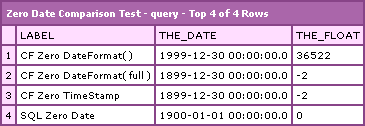Ben Nadel at CFUNITED 2010 (Landsdown, VA) with: David Frazier ( @susqurugger ) Vicky Ryder ( @fuzie ) Saurin Shah ( @vtecnrg )

# Caution: ColdFusion Zero Date vs. SQL Zero Date

By on
Tags: ,

In the past, I have talked a lot about the FLOAT equivalents of date/time stamps in both ColdFusion and SQL. I have talked about how fast they are and how they can be manipulated. But, one thing that I have never really touched upon is how the zero dates in ColdFusion are different from the zero dates in SQL (well, at least how it operates on my server configuration).

Caution! They are different. Not only are they different, but you have to be careful in what format you pass dates to SQL. Take a look at the SQL statement below. I am comparing the SQL zero date to the ColdFusion zero date as well as testing how different DateFormat() calls are converted via the CFQueryParam tag and SQL:

``````<cfquery name="qZeroDate" datasource="...">

<!--- Select the SQL zero date. --->
SELECT
( 'SQL Zero Date' ) AS label,
( CAST( 0.0 AS DATETIME ) ) AS the_date,
( 0.0 ) AS the_float

UNION

<!---
Select the DateFormat() ColdFusion zero date with
--->
SELECT
( 'CF Zero DateFormat( )' ) AS label,
(
<cfqueryparam
value="#DateFormat( 0 )#"
cfsqltype="CF_SQL_TIMESTAMP"
/>
) AS the_date,
CAST(
<cfqueryparam
value="#DateFormat( 0 )#"
cfsqltype="CF_SQL_TIMESTAMP"
/>
AS FLOAT
) AS the_float

UNION

<!---
Select the TimeStamp ColdFusion zero date (ie. Let
CFQueryParam make the conversion for us.
--->
SELECT
( 'CF Zero TimeStamp' ) AS label,
(
<cfqueryparam
value="0"
cfsqltype="CF_SQL_TIMESTAMP"
/>
) AS the_date,
CAST(
<cfqueryparam
value="0"
cfsqltype="CF_SQL_TIMESTAMP"
/>
AS FLOAT
) AS the_float

UNION

<!--- Select the ColdFusion zero date with full date maks. --->
SELECT
( 'CF Zero DateFormat( full )' ) AS label,
(
<cfqueryparam
value="#DateFormat( 0, 'full' )#"
cfsqltype="CF_SQL_TIMESTAMP"
/>
) AS the_date,
CAST(
<cfqueryparam
value="#DateFormat( 0, 'full' )#"
cfsqltype="CF_SQL_TIMESTAMP"
/>
AS FLOAT
) AS the_float
</cfquery>
``````

When I dump out that query, here is what I get:As you can see, the zero date in ColdFusion is two days different from the zero date in MS SQL Server. Now, let me state clearly that I am not sure why this is or if it is just this way on my server. I can only state for a fact that it is this way on MY server. Notice also that the default DateFormat() mask in ColdFusion makes SQL think it is dealing with 1999. That is because the default DateFormat() mask uses a 2-digit year: "d-mmm-yy". When SQL sees the 2-digit year, it assumes we are talking 100 years later than we actually mean (Y2k much?).

So anyway, that is just a word of caution. Date "math" is awesome, I highly recommend it. Just remember that the date math in one universe (CF) doesn't necessarily convert directly to the date math in another universe (SQL).

Want to use code from this post? Check out the license.It's worse than that....
in SQL
Select @curDate = getDate()
Select

easy enough right?
Well apparently SQL knows that 12/31/1899 is 1 quarter further away than 1/1/1900,
Select DATEDIFF(qq,0,@curDate),DATEDIFF(qq,-1,@curDate)

Gives us 443, and 444 quarters difference...

Coldfusion does not...
(lets start at 2, the difference between SQL 0 and CF 0)
#DATEDIFF("q",2,now())#
#DATEDIFF("q", 1, now())#
#DATEDIFF("q", 0, now())#
#DATEDIFF("q", -1, now())#
#DATEDIFF("q", -2, now())#
#DATEDIFF("q", -3, now())#
#DATEDIFF("q", -28, now())#

they all return 443 until we go back 28 days!,
looks like coldfusion is saying "literally" how many quarters of days can fit between the dates... not the actual difference in quarters.